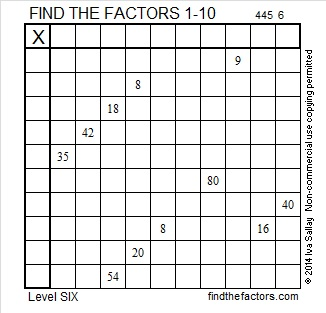# 295 and Level 6

• 295 is a composite number.
• Prime factorization: 295 = 5 x 59
• The exponents in the prime factorization are 1 and 1. Adding one to each and multiplying we get (1 + 1)(1 + 1) = 2 x 2 = 4. Therefore 295 has exactly 4 factors.
• Factors of 295: 1, 5, 59, 295
• Factor pairs: 295 = 1 x 295 or 5 x 59
• 295 has no square factors that allow its square root to be simplified. √295 ≈ 17.176These eleven clues could be pretty tricky!Print the puzzles or type the factors on this excel file: 10 Factors 2014-11-10This site uses Akismet to reduce spam. Learn how your comment data is processed.